# Wolfram|Alpha Makes Easy Work of Any Integer Sequence

January 23, 2012 —Comments Off

What rule gives the integer sequence 3, 10, 17, 18, 7, …? Wolfram|Alpha can easily find that this sequence comes from a simple cubic polynomial, –x3 + 6x2 – 4x + 2.

A different sequence, 1, 1, 3, 7, 22, 82, 333, 1448, … can be identified as the sequence of the polyhexes. After that, the input sequence of the polyhexes recovers the above sequence.

Wolfram|Alpha can recognize millions of different sequences. Rules for the sequence terms are either recognized algorithmically or as part of the On-Line Encyclopedia of Integer Sequences (OEIS), such as 8, 14, 38, 68, 98, 104, 194, 224 ….

Sequences that have been given names historically have many interesting mathematical properties. Wolfram|Alpha gives the notation, description, terms, formula, recurrence relation, ordinary and exponential generating function, a table program, and a plot. The results for the sequence of the tetrahedral numbers are shown here.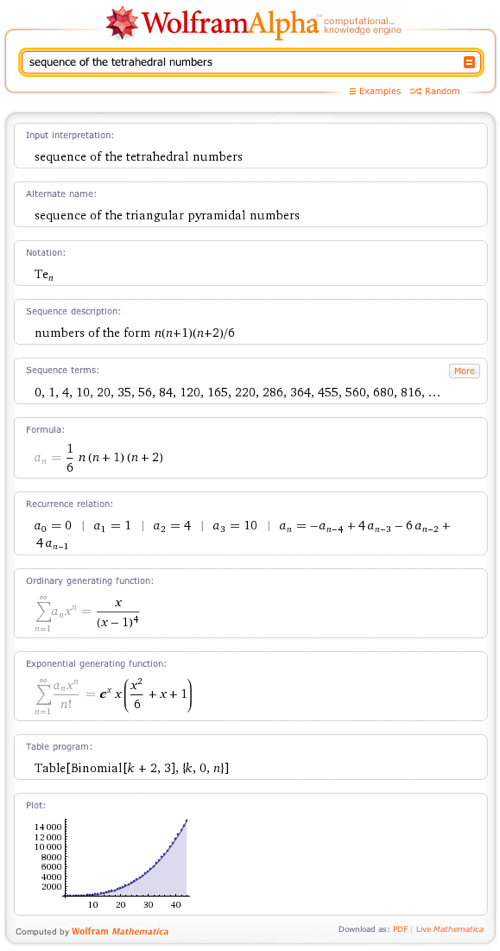If one asks Wolfram|Alpha for a specific property of a sequence, say a generating function, with a query such as generating function of the multichoose sequence, one obtains additional information, such as a plot of the generating function.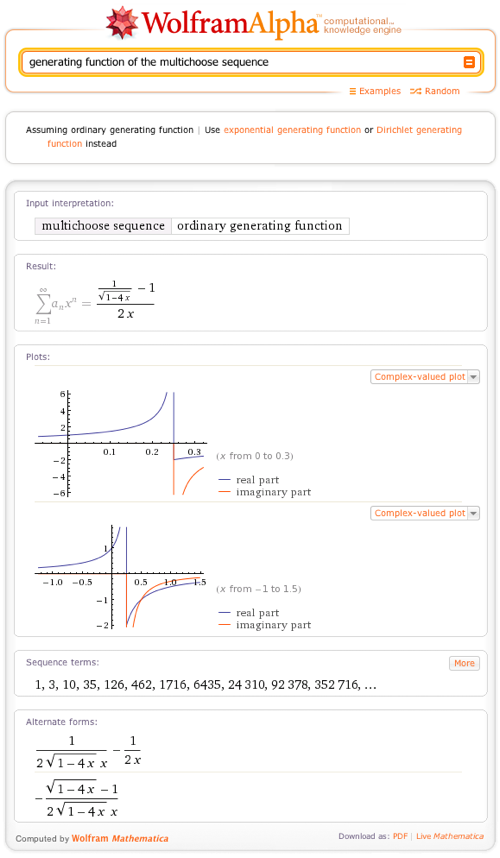Conversely, we can ask Wolfram|Alpha to calculate the series (-1 + 1/Sqrt[1 – 4x])/(2x) at x = 0 to order 12, giving a result that recovers the sequence terms of the multichoose sequence. Another input is the Dirichlet generating function sequence of the tritriangular numbers. The result contains Riemann zeta functions. Specific sequence formulas can also looked at, such as the sequence of the Lah numbers formula.

In addition, many sequences come with recurrence relations, as seen in the sequence of the cubes recurrence relation. The cubes fulfill a linear recurrence relation of order 4. For the recurrence relation without the concrete initial conditions for the cubes, Wolfram|Alpha gives the general solution of the recurrence relation a(n) = –a(n – 4) + 4 a(n – 3) – 6 a(n – 2) + 4 a(n – 1) as a cubic polynomial in n.

Using the CDF-only interactive results with Wolfram|Alpha with CDF allows us to investigate many modular properties of sequences. Even simple sequences, such as the sequence of numbers that are not squares, show unexpected features if the differences are plotted modulo m. All of these results are displayed interactively.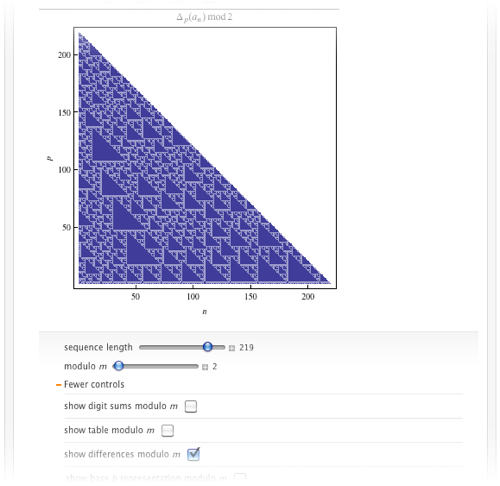While most sequences are just monotonously increasing, some show a rich behavior of going up and down, for example, plot of the sequence of Recamán numbers. Looking up EKG sequence table program also gives Mathematica code for generating the first n terms of the sequence, which can be directly used in Mathematica through the WolframAlpha[] function. For example, here’s a plot of the terms. After 2, each term is the smallest number not already used that shares a factor with the previous term.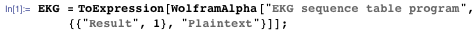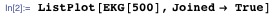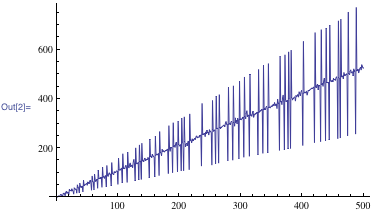Once the sequence terms are available in Mathematica, many more investigations of the sequence are possible. For instance, one can visualize the sequence as a 3D curve.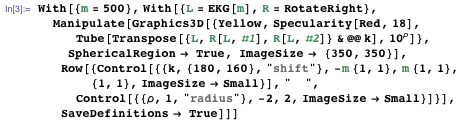[WriCDF source=”https://content.wolfram.com/uploads/sites/35/2012/01/integercdf.cdf” width=”420″ height=”500″ altcont=”To view this content, please install Wolfram CDF Player. You can install the free CDF Player here.”]

We hope that the combination of Mathematica‘s analysis programs and OEIS recognition will prove useful for everyone.